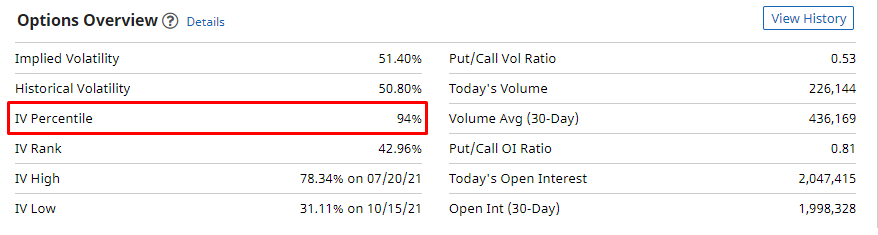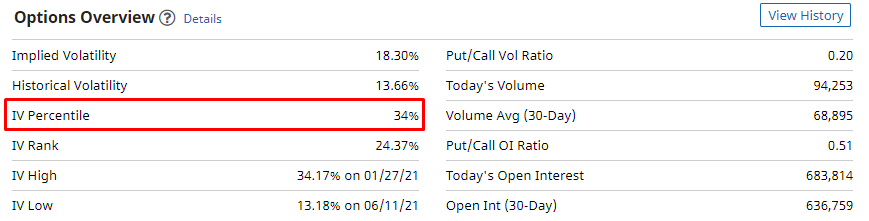When trading options, there is a multitude of data to look at and consider. Some traders focus on support & resistance, indicators, and volume, but Implied Volatility is one of the most critical data points to consider. Knowing what implied volatility (IV) is and how it affects your options trades will dramatically develop your options trading strategy. Even though it might sound complicated, it's actually pretty simple to understand!

How Implied Volatility Is Connected To Options Prices

1. The higher implied volatility is, the more expensive the options will be.
2. The lower implied volatility is, the less expensive the options will be.

Investopedia says that implied volatility is "a metric that captures the market's view of the likelihood of changes in a given security's price. Investors can use implied volatility to project future moves and supply and demand, and often employ it to price options contracts."

Implied volatility is very important and basically represents the options pricing on a given stock. Implied volatility is arguably the most important factor to look at when trading options since it tells you whether the options are cheap or expensive. Implied volatility can be plotted as an indicator, or you can find the value on websites like Barchart.com. Some brokers, like Robinhood, make it very difficult to find the implied volatility on a stock. Luckily, we have a pretty simple solution to this problem.

We will use NVDA stock as an example. If you follow this link, you can see NVDA stock's implied volatility by scrolling down to "Options Overview." You can search and find the implied volatility on any stock using this link and then the search bar at the top.Looking at the chart above, you can see how NVDA stock's implied volatility has been going up a lot recently as the stock started to explode. Many traders were getting greedy with the stock and were speculating on the price to continue to rise. Since so many speculators kept buying up NVDA's options and the stock was very volatile, the prices for NVDA options rose, increasing the implied volatility.

Situations like this can be very profitable for you, but only if you play them correctly. Generally speaking, you want to buy options when implied volatility is low and short options when implied volatility is high. Remember: implied volatility is essentially just a gauge of whether options are cheap or expensive.

If you were to buy options on NVDA while implied volatility was high and then implied volatility fell, your position would lose way more value than usual. Let's say you purchased NVDA calls, and NVDA stock kept rising, but the implied volatility fell. It's likely that you would still lose money even though you predicted the stock direction correctly. This is because you bought the call options when they were expensive and the implied volatility falling significantly reduced the value of the options you bought. Keep in mind that there are A LOT of variables in the options market, and this is just a simple example to illustrate the importance of implied volatility.

Why "IV Percentile" Is So Important

Observing where implied volatility is today relative to where it has been in the past is probably the most important aspect of options trading. Using IV percentile is one way to set yourself up for success. According to Merriam-Webster, percentile is "a value on a scale of 100 that indicates the percent of a distribution that is equal to or below it". How this applies to the options market: If IV percentile is high, 94%, for example, then that means 94% of the time implied volatility is lower than it is right now. This means implied volatility is only this high 6% of the time. It'd be reasonable to say that NVDA's implied volatility is higher than usual and that it might drop soon. You don't want to buy any options when IV Percentile is high because that means the options are expensive.If you search for a stock like WMT on Barchart.com, you will see a lower IV Percentile than NVDA. Way lower actually, 34%.This means that implied volatility is higher than the current level 66% of the time. You could also say that implied volatility is only lower than the current level 34% of the time. So, in this case, it would mean WMT has a lower implied volatility than usual right now. This would also mean that WMT's options are priced pretty well right now.

Why Is All Of This So Important?

Our new Advanced Options course has a TON of perks!!